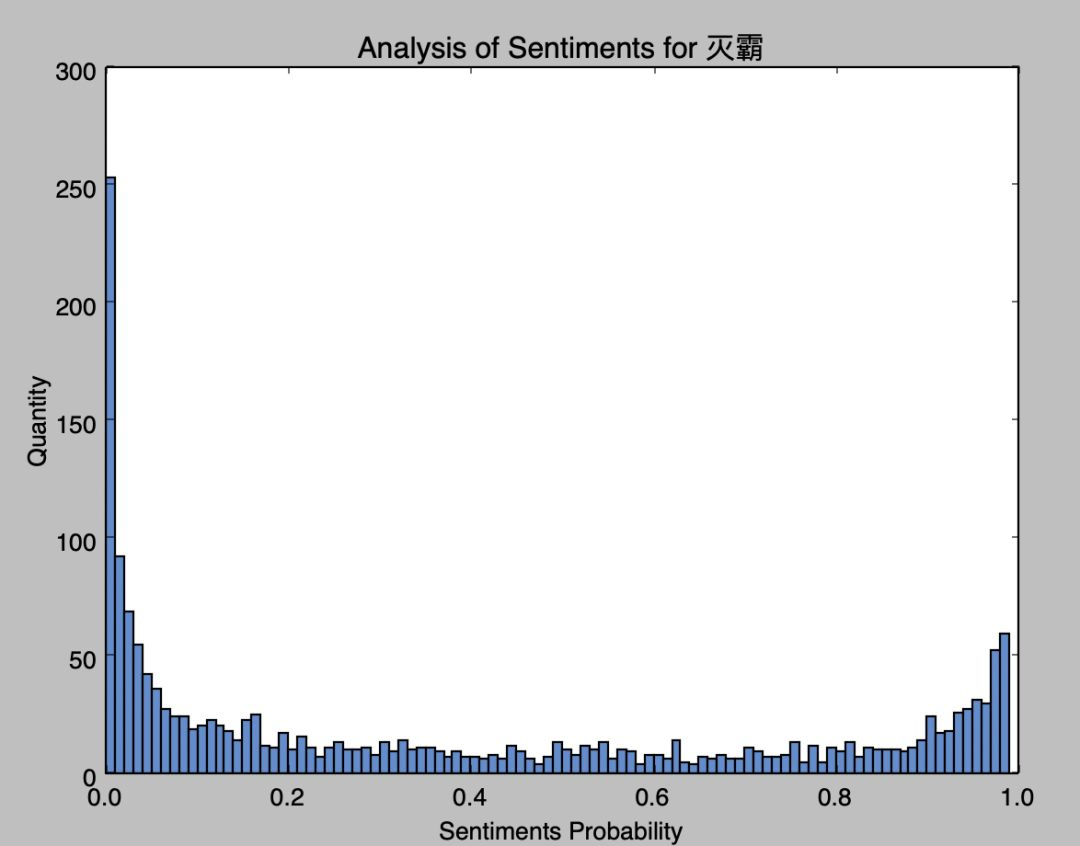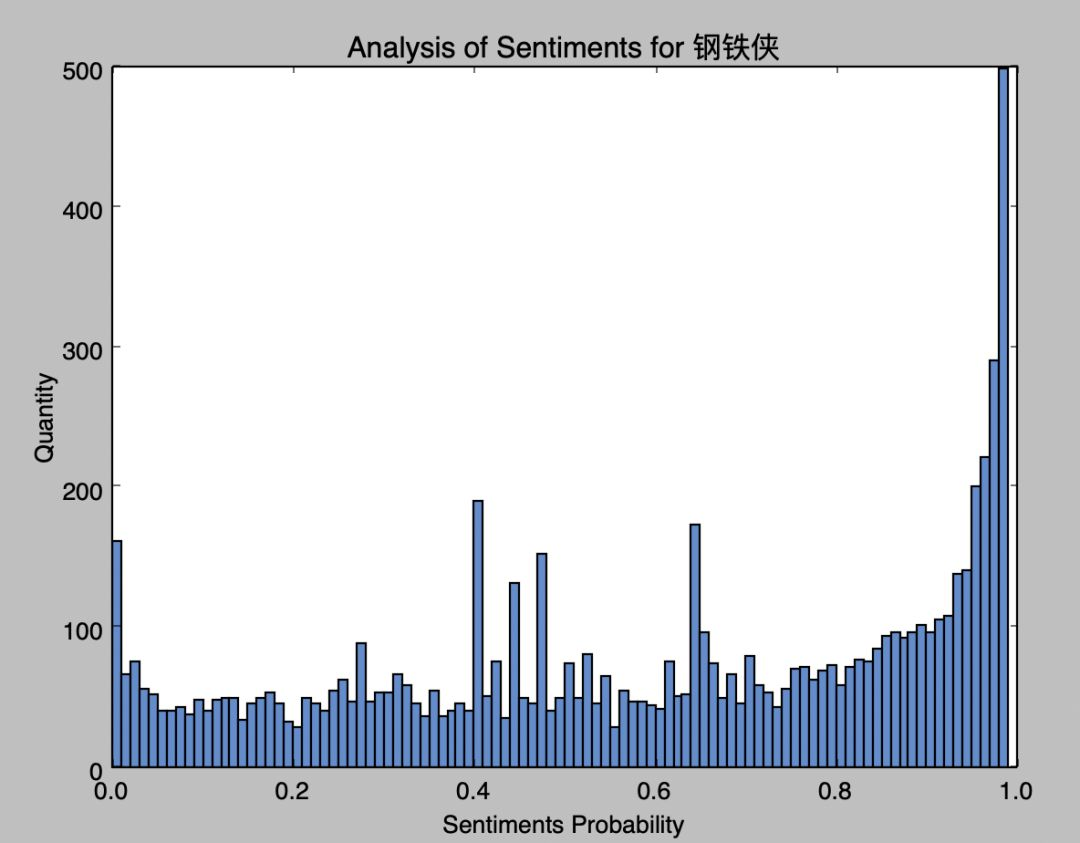# Python 分析 9 萬條資料告訴你復仇者聯盟誰才是絕對 C 位！《復聯 4》國內上映第十天，程式設計師的江湖裡開始流傳這樣一個故事，即：

—— 以上來自知乎@郭啟軍

http://m.maoyan.com/mmdb/comments/movie/ 248172.json?_v_=yes&offset= 20&startTime= 2019-04-24% 2002: 56: 46

defgetMoveinfo(url):

session = requests.Session()

“User-Agent”: “Mozilla/5.0”,

“Accept”: “text/html,application/xhtml+xml”,

}

ifresponse.status_code == 200:

returnresponse.text

returnNone

defsaveitem(dbName, moveId, id, originaldata):

conn = sqlite3.connect(dbName)

conn.text_factory=str

cursor = conn.cursor()

ins= “INSERT OR REPLACE INTO comments values (?,?,?)”

v = (id, originalData, moveId)

cursor.execute(ins,v)

cursor.close()

conn.commit()

conn.close()

defconvert(dbName):

conn = sqlite3.connect(dbName)

conn.text_factory = str

cursor = conn.cursor()

data = cursor.fetchall()

foritem indata:

movieId = item[ 2]

insertItem(dbName, movieId, commentItem)

cursor.close()

conn.commit()

conn.close()

definsertItem(dbName, movieId, item):

conn = sqlite3.connect(dbName)

conn.text_factory = str

cursor = conn.cursor()

sql = ”’

INSERT OR REPLACE INTO convertData values(?,?,?,?,?,?,?,?,?)

”’

values = (

getValue(item, “id”),

movieId,

getValue(item, “userId”),

getValue(item, “nickName”),

getValue(item, “score”),

getValue(item, “content”),

getValue(item, “cityName”),

getValue(item, “vipType”),

getValue(item, “startTime”))

cursor.execute(sql, values)

cursor.close()

conn.commit()

conn.close()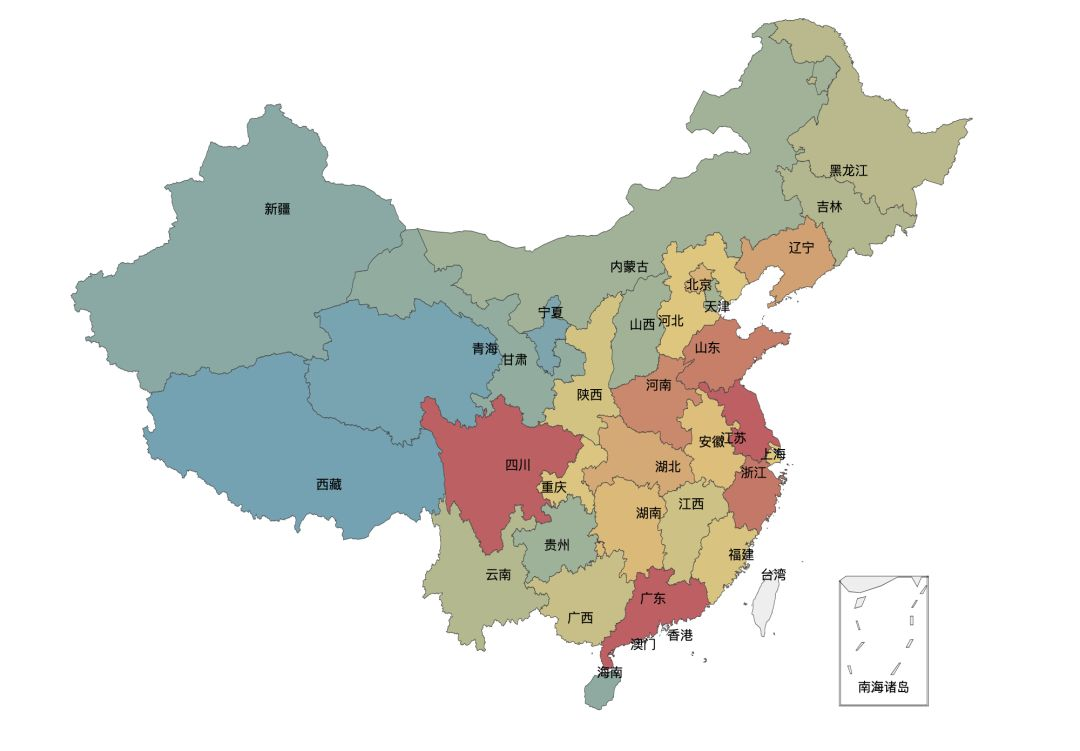data = pd.read_sql( “select * from convertData”, conn)

city = data.groupby([ ‘cityName’])

city_com = city[ ‘score’].agg([ ‘mean’, ‘count’])

city_com.reset_index(inplace= True)

fo = open( “citys.json”, ‘r’)

printcity_com

data_map_all = [(getRealName(city_com[ ‘cityName’][i], citysJson),city_com[ ‘count’][i]) fori inrange( 0,city_com.shape[ 0])]

data_map_list = {}

foritem indata_map_all:

ifdata_map_list.has_key(item[ 0]):

value = data_map_list[item[ 0]]

value += item[ 1]

data_map_list[item[ 0]] = value

else:

data_map_list[item[ 0]] = item[ 1]

data_map = [(realKeys(key), data_map_list[key] ) forkey indata_map_list.keys()]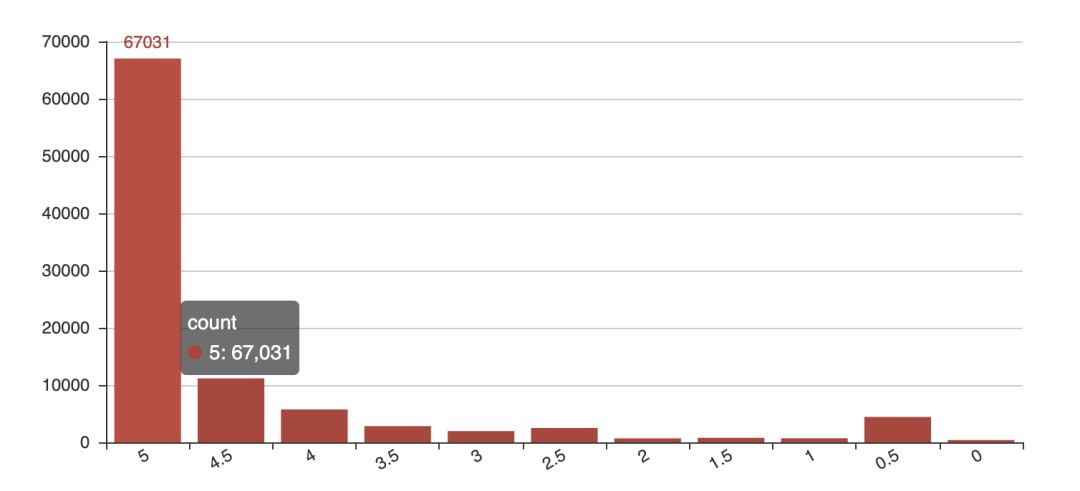attr = [ “滅霸”, “美國隊長”,

“鋼鐵俠”, “浩克”, “奇異博士”, “蜘蛛俠”, “索爾”, “黑寡婦”,

“鷹眼”, “驚奇隊長”, “幻視”,

“猩紅女巫”, “蟻人”, “古一法師”]

alias = {

“滅霸”: [ “滅霸”, “Thanos”],

“美國隊長”: [ “美國隊長”, “美隊”],

“浩克”: [ “浩克”, “綠巨人”, “班納”, “HULK”],

“奇異博士”: [ “奇異博士”, “醫生”],

“鋼鐵俠”: [ “鋼鐵俠”, “stark”, “斯塔克”, “託尼”, “史塔克”],

“蜘蛛俠”: [ “蜘蛛俠”, “蜘蛛”, “彼得”, “荷蘭弟”],

“索爾”:[ “索爾”, “雷神”],

“黑寡婦”: [ “黑寡婦”, “寡姐”],

“鷹眼”:[ “鷹眼”, “克林頓”, “巴頓”, “克林特”],

“驚奇隊長”:[ “驚奇隊長”, “卡羅爾”, “驚奇”],

“星雲”:[ “星雲”],

“猩紅女巫”: [ “猩紅女巫”, “緋紅女巫”, “旺達”],

“蟻人”:[ “蟻人”, “蟻俠”, “Ant”, “AntMan”],

“古一法師”: [ “古一”, “古一法師”, “法師”]

}

v1 = [getCommentCount(getAlias(alias, attr[i])) fori inrange( 0, len(attr))]

bar = Bar( “Hiro”)

xaxis_interval= 0,is_splitline_show= True)

bar.render( “html/hiro_count.html”)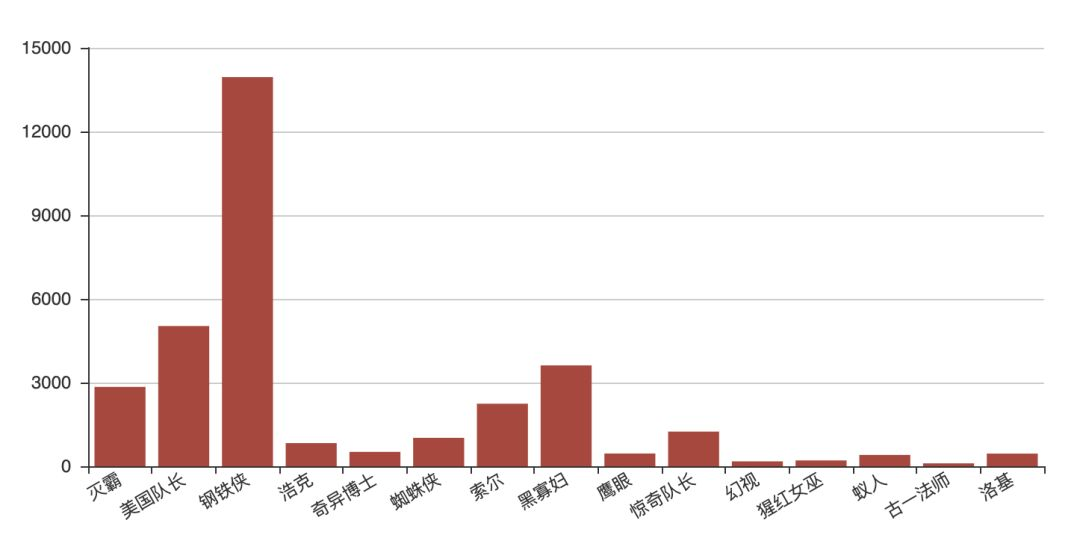defemotionParser(name):

conn = conn = sqlite3.connect( “end.db”)

conn.text_factory = str

cursor = conn.cursor()

likeStr = “like “%”+ name + “%””

cursor.execute( “select content from convertData where content “+ likeStr)

values = cursor.fetchall()

sentimentslist = []

foritem invalues:

sentimentslist.append(SnowNLP(item[ 0].decode( “utf-8”)).sentiments)

plt.hist(sentimentslist, bins=np.arange( 0, 1, 0.01), facecolor= “#4F8CD6”)

plt.xlabel( “Sentiments Probability”)

plt.ylabel( “Quantity”)

plt.title( “Analysis of Sentiments for “+ name)

plt.show()

cursor.close()

conn.close()